# How to Multiply in Excel — 4 Easy Solutions

Posted April 11, 2019 by Sheraz Ali in Microsoft Excel

You can use many different methods to multiply in excel. They are quite similar to the protocols used for subtraction, addition, and division. Each has its own use. Some are suitable for multiplying within single cells, whereas others are used for multiplying an entire range or column of cells.

In this tutorial, we will teach you how to multiply in excel in the easiest way possible, with simple visual instructions.

## Step-by-Step: How to Multiply in Excel

### Method 1: Multiplying in a Single Cell

1. Open your Excel Spreadsheet.

2. Click on an Empty Cell.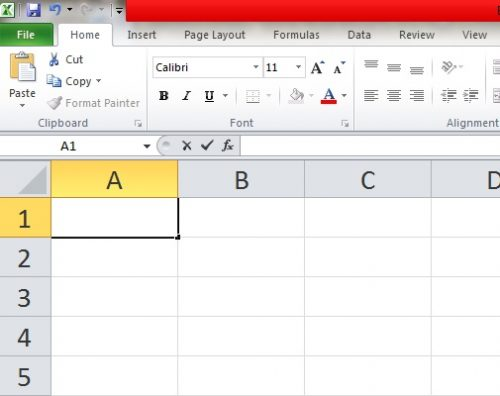3. Start your formula with a “=”.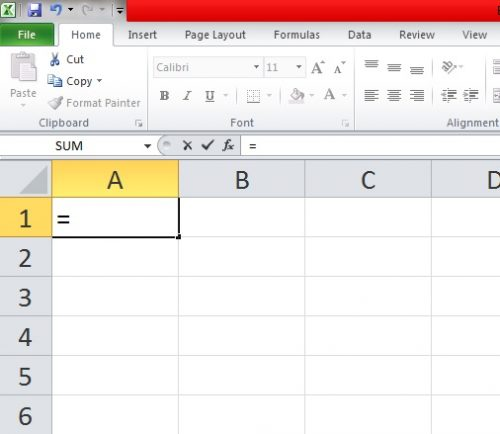4. Type the numbers you want to multiply, separated by an asterisk “*” sign.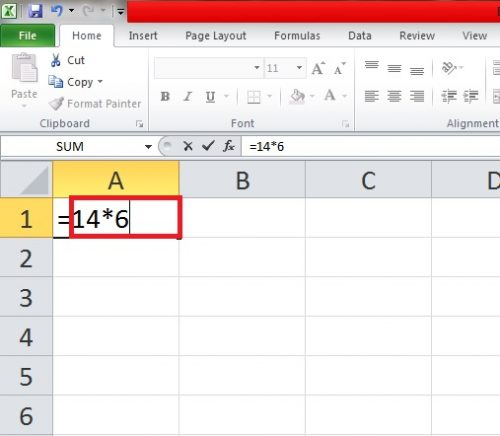5. Hit Enter.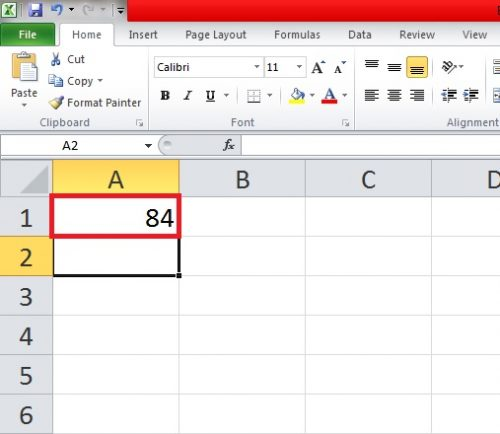### Method 2: Multiplying a Column or an Entire Range of Cells

1. Open the Excel Spreadsheet.

2. Insert your Data.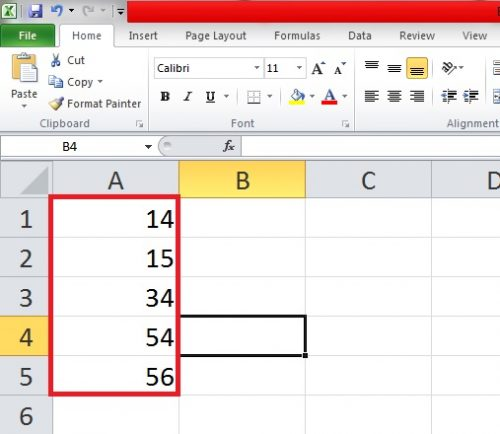3. Click an empty Cell.

4. Start your formula with a “=” sign.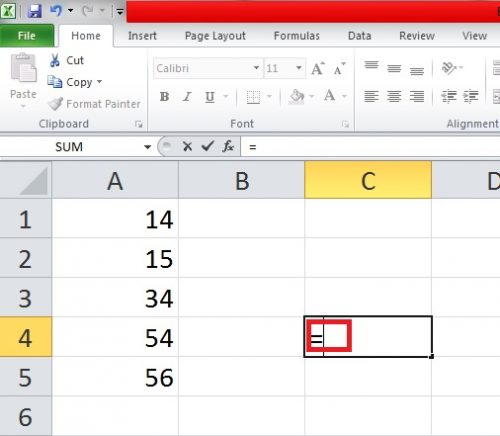5. Type the Product formula followed by a bracket: =Product(.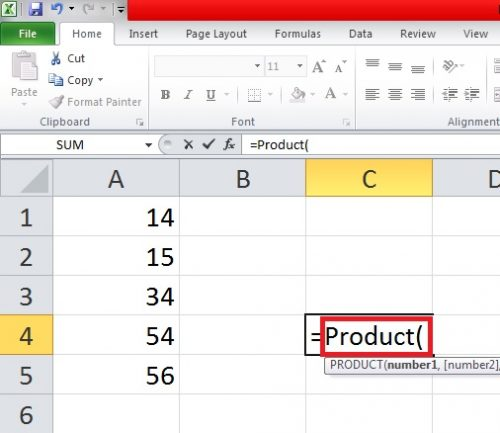6. Select the cells you want to multiply.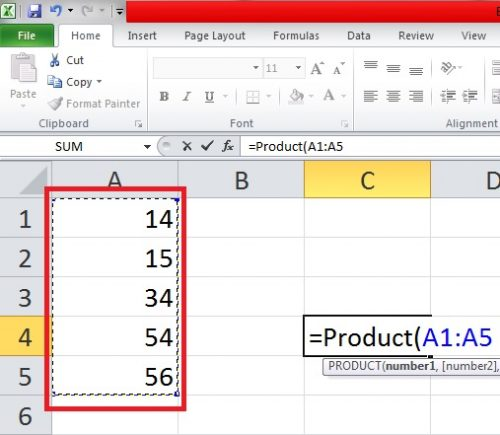7. Hit Enter.

### Method 3: Multiplying two Columns of Cells

1. Open your Excel Spreadsheet.

2. Insert your data.

3. Click cell C1 and start your formula with an equal (=) sign.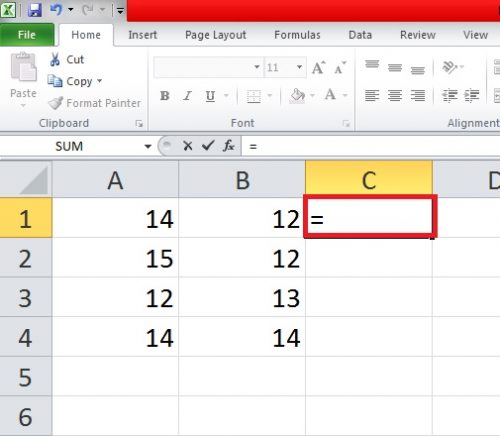4. Simply multiply cells A1 and B1 like this: A1*B1.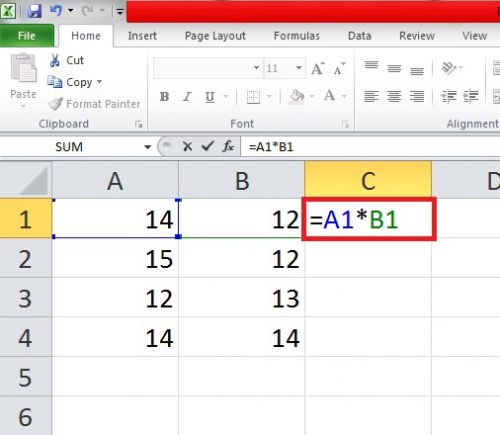5.Hit Enter.

6. Stretch the answer by dragging it down to the rest of rows or cells. Excel will automatically multiply them.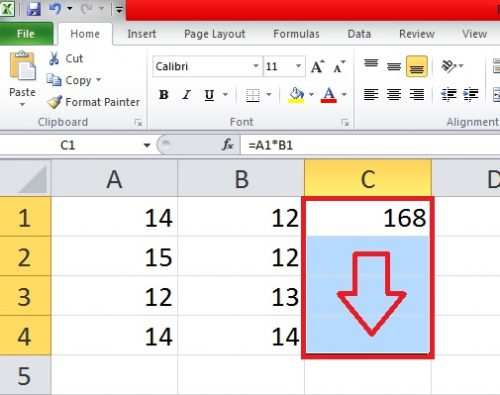### Method 4: Multiplying with a Constant Number

To multiply with a constant number, insert a dollar sign (\$) before the asterisk sign.

Your formula will look like this in this case: A1*\$A\$5.

This is how to multiply in excel with unflinching ease. It doesn’t take a minute and makes things a hell of a lot easier for you. You can choose to manually multiply a range of cells but it will only make things harder and waste precious time.

If you have any questions or queries, talk to us. We want to hear from you. We will try our best to answer.

For additional guidance, you can check this related tech-recipe: how to add-drop down list in excel

You can also check our FAQ if you still have yourself riled in intricacies and confusions.

### Frequently Asked Questions

Can I multiply two or numbers in the older version of Microsoft Excel with the same methods?

Yes, you can. These steps are applicable to any version of Excel, right down to the 2003 version.

What formula should I use to perform multiplication in excel?

The formula for multiplication in excel is =Product(.

What if I have to multiply with the same number in Excel?

Check the Method No.4 for this question.

### About Sheraz Ali

An established copywriter, with a longstanding experience in a vast array of industries, including but not limited to spirituality, technology, cannabis and travel.
View more articles by Sheraz Ali

#### The Conversation

Follow the reactions below and share your own thoughts.